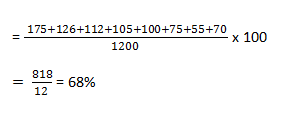New Students Offer - Use Code HELLO

# Data Analysis Set for IBPS PO Mains: Part 9Directions (1-7): Study the following information carefully and answer the questions given below:

In an engineering college, there are 2500 students studying Mechanical and Civil Engineering. Each student from each course knows one or more languages out of English, Hindi and German. 48% of
the students study Mechanical Engineering. Out of the students studying Mechanical Engineering. Out of the students studying Mechanical Engineering, boys and girls are in the ratio of 7:5. Out of the boys studying Mechanical Engineering 12% know only English, 9% know only Hindi and 5% know only German. 25% know English as well as Hindi. 18% know English as well as German. 16% know Hindi as well as German. Remaining boys knows all three languages.

Out the girls studying Mechanical Engineering, 18% know only English, 10% know only Hindi and 12% know only German. 20% know English as well as Hindi. 15% know English as well as German. 11% know Hindi as well as German. remaining girls know all three languages.

Out of the students studying Civil, boys and girls are in the ratio of 5:8 Out of the boys studying Civil, 18% know only English, 14% know only Hindi. 26% know English as well as German. 12% know Hindi as well as German. Remaining boys knows all three languages.

Out of the girls studying Civil Engineering, 14% know only English, 15% know only Hindi and 6% know only German, 22% know English as well as Hindi. 21% know English as well as German, 20% know Hindi as well as German. Remaining girls know all three languages.

1. Out of the total number of students studying of studying Mechanical Engineering, approximately what percent of students know at least two languages?
1. 62%
2. 64%
3. 65%
4. 68%
5. 70%

2. How many students in the college know all three languages?
1. 226
2. 126
3. 275
4. 190
5. 210

3. Out of the students studying Civil Engineering, what is the ratio the number of boys knowing English to the number of girls knowing Hindi?
1. 335:473
2. 337:472
3. 336:473
4. 335:474
5. 335:472

4. What percent of the total number of girls knows Engineering know either only Hindi or only English?
1. 198
2. 287
3. 267
4. 256
5. 273

5. How many students studying Mechanical Engineering know either only Hindi or only English?
1. 198
2. 287
3. 267
4. 256
5. 273

6. How many students studying Civil Engineering, do not know English?
1. 483
2. 493
3. 455
4. 475
5. 465

7. What percent of the total number of girls in the college do not know Hindi?
1. 42.54%
2. 38.54%
3. 34.68%
4. 44.98%
5. 47.68%### Mechanical Engineering :

Number of boys = 700
Number of girls = 500

Boys Knowing :
Only English = 12 x 7 = 84
Only Hindi = 9 x 7 = 63
Only German = 5 x 7 = 35
English + Hindi = 25 x 7 = 175
English + German = 18 x 7 = 126
German + Hindi = 16 x 7 = 112
English + Hindi + German = 105

Girls Knowing:
Only English = 18 x 5 = 90
Only Hindi = 10 x 5 = 50
Only German = 12 x 5 = 60
English + Hindi = 20 x 5 = 100
English + German = 15 x 5 = 75
German + Hindi = 11 x 5 = 55
English + Hindi + German = 70

### Civil Engineering :

Number of boys = 500
Number of girls = 800

Boys Knowing :
Only English = 18 x 5 = 90
Only Hindi = 14 x 5 = 70
Only German = 7 x 5 = 35
English + Hindi = 16 x 5 = 80
English + German = 12 x 5 = 60
Hindi + German = 12 x 5 = 60
English + Hindi + German = 35

Girls Knowing :
Only English = 14 x 8 = 112
Only HIndi = 15 x 8 = 120
Only German = 6 x 8 = 48
English + Hindi = 22 x 8 = 176
English + German = 21 x 8 = 168
Hindi + German = 20 x 8 = 160
Hindi + English + German = 16

1. 4;
Required percent2. 1;
Required answer = 105 + 70 + 35 + 16 = 226

3. 5;
Required ratio
= ( 90 + 80 + 130 + 35) : ( 120 + 176 + 160 + 16)
= 335 : 472

4. 3;
Required percent5. 2;
Required answer = 84 + 63 + 90 + 50 = 287

6. 2;
Required answer = 70 + 35 + 60 + 120 + 48 + 160 = 493

7. 1;
Required percent